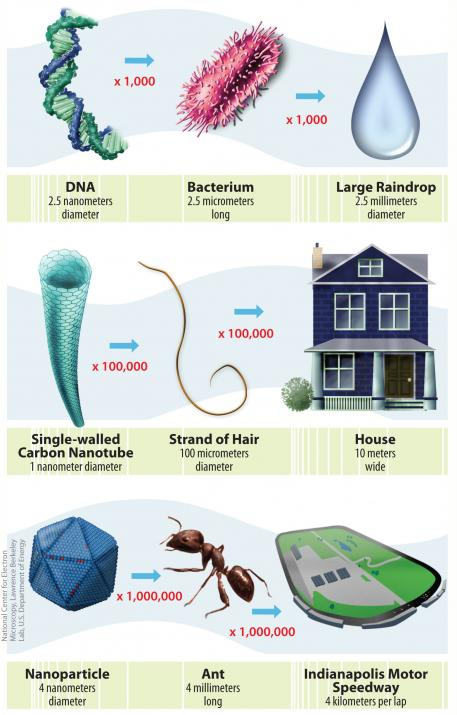# Size of the Nanoscale

Just how small is “nano?” In the International System of Units, the prefix "nano" means one-billionth, or 10-9; therefore one nanometer is one-billionth of a meter. It’s difficult to imagine just how small that is, so here are some examples:

• A sheet of paper is about 100,000 nanometers thick
• A strand of human DNA  is 2.5 nanometers in diameter
• There are 25,400,000 nanometers in one inch
• A human hair is approximately 80,000- 100,000 nanometers wide
• A single gold atom is about a third of a nanometer in diameter
• On a comparative scale, if the diameter of a marble was one nanometer, then diameter of the Earth would be about one meter
• One nanometer is about as long as your fingernail grows in one second

The illustration below has three visual examples of the size and the scale of nanotechnology, showing just how small things at the nanoscale actually are.The Scale of Things